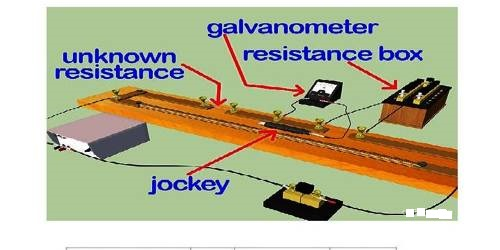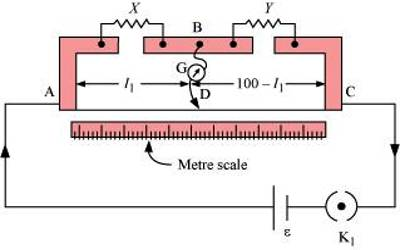Physics

# Meter BridgeMeter bridge: The instrument in which having uniform section of lm the resistance of a wire of uniform cross-section of lm length is used and following Wheatstone bridge principle an unknown resistance can be determined is called a meter bridge.

A meter bridge is an apparatus used to find the resistance of a coil; you will find it as part of the tools of a physics lab. It is a Wheatstone bridge in which one lateral pair of resistances is replaced by a straight wire of length 1m of the uniform cross section. And other pair consists of one known and one unknown resistance. One side of a galvanometer is connected between known and unknown resistance and another side is moved along the wire to find the null point i.e. at which galvanometer doesn’t show any deflection. This point balances the bridge.A meter bridge is a simple type of potentiometer which may be used in school science laboratories to demonstrate the principle of resistance measurement by potentiometric means. A resistance wire is laid along the length of a meter rule and contact with the wire is made through a galvanometer by a slider. When the galvanometer reads zero, the ratio between the lengths of wire to the left and right of the slider is equal to the ratio between the values of a known and an unknown resistor in a parallel circuit.